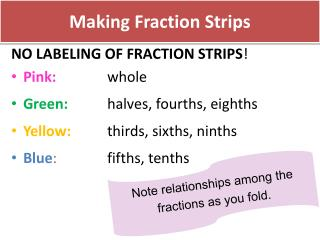Download PresentationMaking Fraction Strips# Making Fraction Strips - PowerPoint PPT Presentation

Download Presentation##### Making Fraction Strips

Download Policy: Content on the Website is provided to you AS IS for your information and personal use and may not be sold / licensed / shared on other websites without getting consent from its author. While downloading, if for some reason you are not able to download a presentation, the publisher may have deleted the file from their server.

- - - - - - - - - - - - - - - - - - - - - - - - - - - E N D - - - - - - - - - - - - - - - - - - - - - - - - - - -
##### Presentation Transcript

1. Making Fraction Strips NO LABELING OF FRACTION STRIPS! • Pink: whole • Green:halves, fourths, eighths • Yellow: thirds, sixths, ninths • Blue:fifths, tenths Note relationships among the fractions as you fold.

2. Apply and Extending Previous Understanding to Operate with Fractions Academic Coaches – Math Meeting February 15, 2013 Beth Schefelker Bridget Schock Connie Laughlin Hank Kepner Kevin McLeod

3. Closest to Half Turn and Talk: Use the task “Closest to Half” from February 1st professional practice. What understandings or misconceptions surfaced in your student work? 3

5. Learning Intentions & Success Criteria Learning Intention We are learning to understand addition as combining and subtraction as the inverse. Success Criteria We will be successful when we can explain why the operations of addition and subtract are the same for fractions as they are for whole numbers.

6. …Apply and Extend previous understanding

7. Bridget’s Week 1 Run Bridget is training to run a marathonby fall. She starts by running 4 miles on Monday and 5 mileson Friday. How many milesdid she run?

8. Bridget’s Week 2 Run Because of the snow, she was only able to run of a mile on Monday and of a mile on Friday. How many miles did she run? Use visual fraction models to help explain your reasoning.

9. 4.NF.3a & b Build fractions from unit fractions by applying and extending previous understandings of operations on whole numbers. 3. Understand a fraction a/b with a>1 as a sum of fractions 1/b. • Understand addition and subtraction of fractions as joining and separating parts referring to the same wholes. • Decompose a fraction into a sum of fractions with the same denominator in more than one way, recording each decomposition by an equation. Justify decomposition by using a visual fraction model.

10. Extending Thinking to 4.NF.3b How many different ways can you decompose the number: 9 Think-Pair-Share How many different ways can you decompose the number: Think-Pair-Share

11. 4.NF.3c & d Build fractions from unit fractions by applying and extending previous understandings of operations on whole numbers. 3. Understand a fraction a/b with a>1 as a sum of fractions 1/b. c. Add and subtract mixed numbers with like denominators, e.g., by replacing each mixed number with an equivalent fraction, and/or by using properties of operations and the relationship between addition and subtraction. d. Solve word problems involving addition and subtraction of fractions referring to the same whole and having like denominators, e.g., by using visual fraction models and equations to represent the problem.

12. Which Operation? • Work in pairs. • Take turns flipping a card and reading the problem. • Use your white boards to write an expression that represents the problem. • Keep track of problems that raised interesting discussions. • Discuss these problems as a table group.

13. Progressions Document • Read p. 6-7 “Adding and Subtracting Fractions” (stop on p.7 at “repeated reasoning…”). • Highlight connections to today’s session.

14. Discuss your understanding • “The meaning of addition is the same for both fractions and whole numbers, even though algorithms for calculating their sums can be different.” • “It is not necessary to know how much 2/3 + 8/5 is exactly in order to know what the sum means.” • “Converting a mixed number to a fraction should not be viewed as a separate technique to be learned by rote, but simply as a case of fraction addition.”

15. Learning Intentions & Success Criteria Learning Intention We are learning to understand addition as combining and subtraction as the inverse. Success Criteria We will be successful when we can explain why the operations of addition and subtract are the same for fractions as they are for whole numbers.

16. Reflection As we’ve progressed in our study of Fractions, in what way did today’s session build on our knowledge of unit fractions and exemplify MP7. Look for and make use of structure?